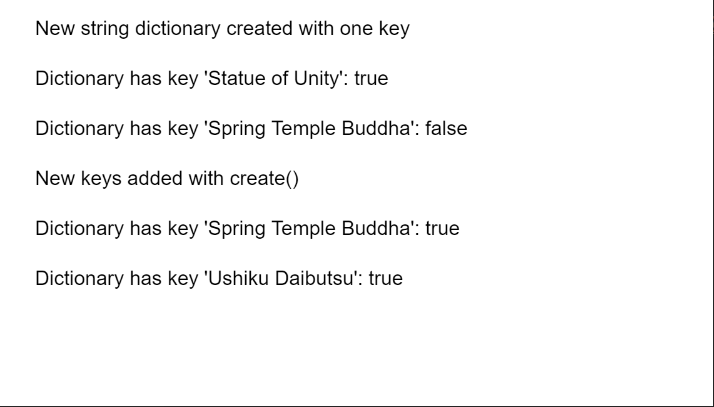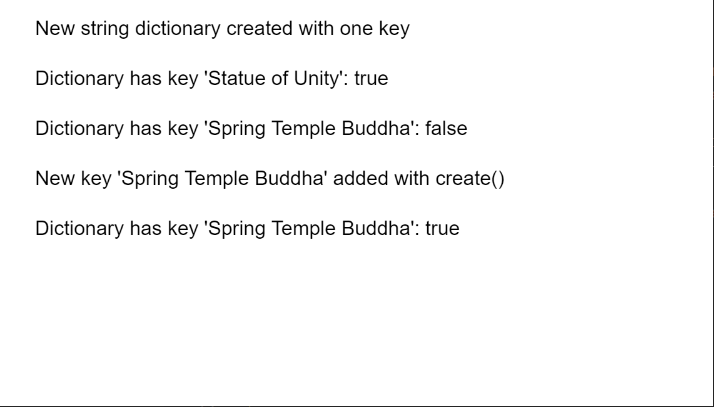GeeksforGeeks App
Open AppBrowser
Continue

# p5.js TypedDict create() Method

The create() method of p5.TypedDict in p5.js is used to add the given key-value pair or collection of pairs to the dictionary. A key-value pair is a set of two values that are mapped to each other. These values can be accessed by querying this dictionary using the key portion of the pair. A dictionary can store multiple key-value pairs that can be accessed using the methods of the dictionary.

Syntax:

```create( key, value )
```

or

```create( obj )
```

Parameters:

• key: It specifies the string that is used as the key to be added to the dictionary.
• value:It specifies the string that is used as the value to be added to the dictionary.
• obj: It specifies the object that contains the key-value pairs to be added to the dictionary.

The example below illustrates the create() method in p5.js:

Example 1:

## Javascript

 `function` `setup() {``  ``createCanvas(550, 300);``  ``textSize(16);`` ` `  ``let stringDict =``      ``createStringDict(``"Statue of Unity"``, ``"182 m"``);``  ``text(``"New string dictionary created "` `+``       ``"with one key"``, 20, 20);`` ` `  ``let existOne = ``      ``stringDict.hasKey(``"Statue of Unity"``);``  ``text(``"Dictionary has key "` `+``       ``"'Statue of Unity': "` `+``       ``existOne, 20, 60);`` ` `  ``let existTwo = ``      ``stringDict.hasKey(``"Spring Temple Buddha"``);``  ``text(``"Dictionary has key "` `+``       ``"'Spring Temple Buddha': "` `+``       ``existTwo, 20, 100);`` ` `  ``let tmpObj = {``    ``"Spring Temple Buddha"``: ``"128 m"``,``    ``"Ushiku Daibutsu"``: ``"100 m"``,``    ``"Great Buddha of Thailand"``: ``"92m"``  ``};`` ` `  ``// Add the given key to the dictionary``  ``// specifying the key and value as an object``  ``stringDict.create(tmpObj);``  ``text(``"New keys added with create()"``,``       ``20, 140);`` ` `  ``existTwo = ``    ``stringDict.hasKey(``"Spring Temple Buddha"``);``  ``text(``"Dictionary has key "` `+``       ``"'Spring Temple Buddha': "` `+``       ``existTwo, 20, 180);`` ` `  ``let existThree =``      ``stringDict.hasKey(``"Ushiku Daibutsu"``);``  ``text(``"Dictionary has key "` `+``       ``"'Ushiku Daibutsu': "` `+ ``       ``existThree, 20, 220);``}`

Output:Example 2:

## Javascript

 `function` `setup() {``  ``createCanvas(550, 300);``  ``textSize(16);`` ` `  ``let stringDict =``      ``createStringDict(``'Statue of Unity'``,``                       ``'182 m'``);``  ``text(``"New string dictionary "` `+``       ``"created with one key"``, 20, 20);`` ` `  ``let existOne =``      ``stringDict.hasKey(``'Statue of Unity'``);``  ``text(``"Dictionary has key 'Statue of Unity': "``        ``+ existOne, 20, 60);`` ` `  ``let existTwo =``      ``stringDict.hasKey(``'Spring Temple Buddha'``);``  ``text(``"Dictionary has key "` `+``       ``"'Spring Temple Buddha': "` `+``       ``existTwo, 20, 100);`` ` `  ``// Add the given key to the dictionary``  ``// specifying the key and value``  ``stringDict.create(``'Spring Temple Buddha'``,``                    ``'128 m'``);``  ``text(``"New key 'Spring Temple Buddha'"` `+``       ``" added with create()"``, 20, 140)`` ` `  ``existTwo = ``    ``stringDict.hasKey(``'Spring Temple Buddha'``);``  ``text(``"Dictionary has key "` `+``       ``"'Spring Temple Buddha': "` `+``       ``existTwo, 20, 180);``}`

Output:Online editor: https://editor.p5js.org/
Environment Setup: https://www.geeksforgeeks.org/p5-js-soundfile-object-installation-and-methods/
Reference: https://p5js.org/reference/#/p5.TypedDict/create

My Personal Notes arrow_drop_up
Related Tutorials